The Electron Gas Current Response Function

Hong Yao June 15, 2008

(Submitted as coursework for Physics 372, Stanford University, Spring 2008)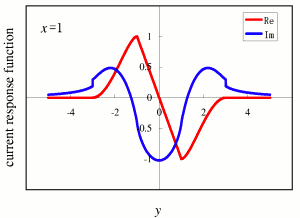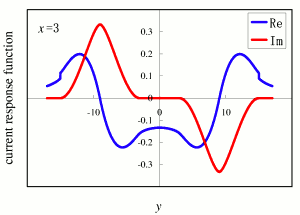Fig. 1: The dimensionless part of the transverse current response function. (a) Both real (blue solid) and imaginary (red solid) parts of the response function are plotted for x=1. (b)Both real (blue dashed) and imaginary parts (red dashed) of the response function are plotted for x=3. The computer code that generated this plot is available here.

In this study, we investigate the free electron gas current response function. The charge current operator is defined as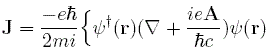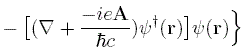where A is the vector potential and &psi(r) is the electron annihilation operator at r. Note that this definition of the current operator above is gauge invariant. We obtain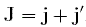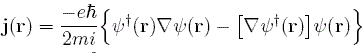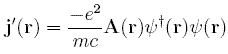Now, we are ready to calculation the current response function. In linear response regime, the perturbative Hamiltonian is given by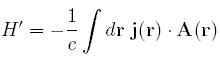where the Coulomb gauge is selected and the A2 term is neglected. Note that in H' it is j, not J, that couples to A. In terms of ck's, which are defined as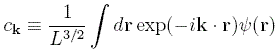the currect j is given by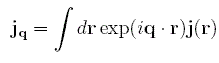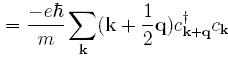Thus, we are ready to obtain the current response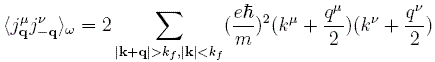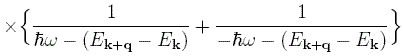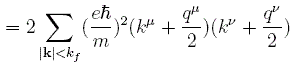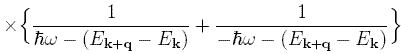where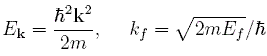and Ef is the Fermi energy of the electron gas.

Due to the spherical symmetry of the electron gas, the response function can be decomposed into longitudinal and transverse parts: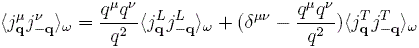The longitudinal part is related to the density response by virtue of the current continuity equation since the particle number is conserved. The continuity equation in the Coulomb gauge is given by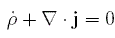which indicates that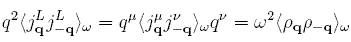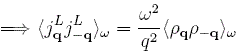Since in the previous report the density response function has been computed, we here only need to compute the transverse part of the current response function as follows: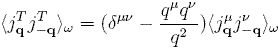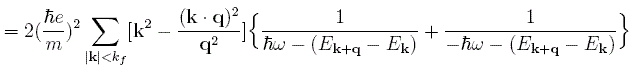whose imaginary part can be evaluated in a closed form. We denote that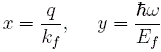Thus, the transverse response function is given by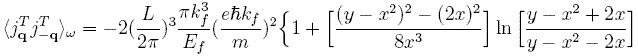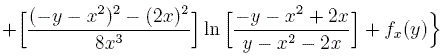where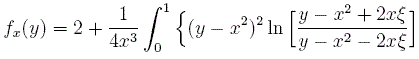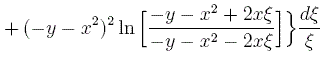This complicated integral has not closed form. However, its imaginary part is simple: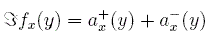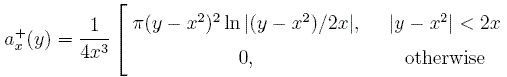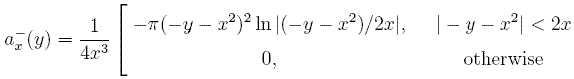Thus, the imaginary part of the transverse current response function is given by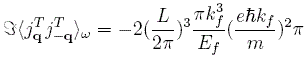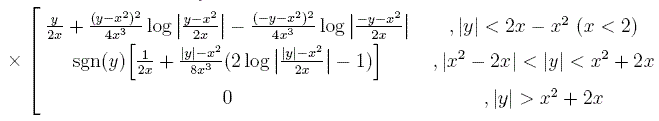Once we obtain the imaginary part of the transverse response function, its real part can be obtained by the Kramers-Kronig relation: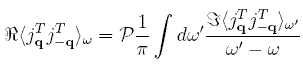where P indicates the principle part of the integral.

© 2008 H. Yao. The author grants permission to copy, distribute and display this work in unaltered form, with attribution to the author, for noncommercial purposes only. All other rights, including commercial rights, are reserved to the author.

References

 J. R. Schrieffer, Theory of Superconductivity (Perseus, 1999).

 A. L. Fetter and J. D. Walecka, Quantum Theory of Many-Particle Systems (Dover, 2003).

 X.-G. Wen, Quantum Field Theory of Many-body Systems (Oxford, 2007).